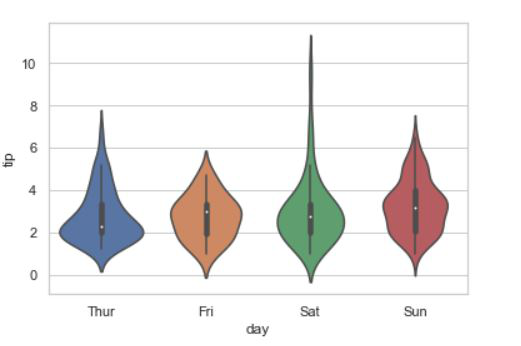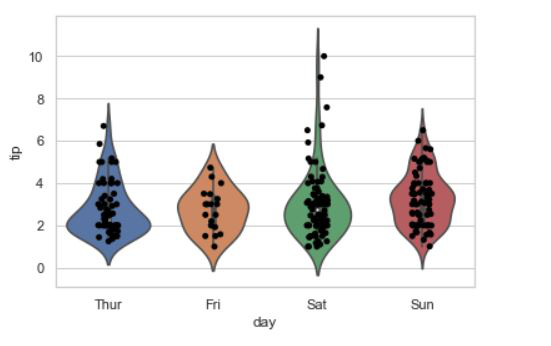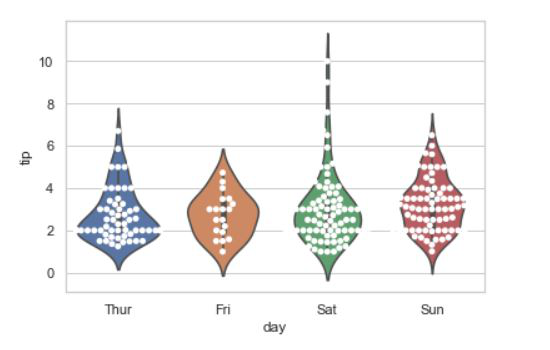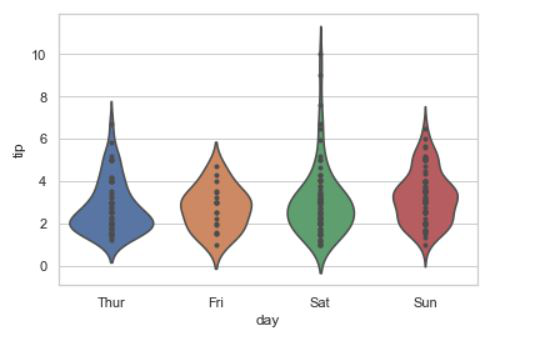Related Articles
How To Make Violinpot with data points in Seaborn?
• Last Updated : 23 Dec, 2020

A violin plot plays a similar activity that is pursued through whisker or box plot do. As it shows several quantitative data across one or more categorical variables. It can be an effective and attractive way to show multiple data at several units. A “wide-form” Data Frame helps to maintain each numeric column which can be plotted on the graph. It is possible to use NumPy or Python objects, but pandas objects are preferable because the associated names will be used to annotate the axes. In this article, we are going to see how to make violinplot with data points.

Syntax: seaborn.violinplot(x=None, y=None, hue=None, data=None, **kwargs)

Parameters:
x, y, hue: Inputs for plotting long-form data.
data: Dataset for plotting.
scale: The method used to scale the width of each violin.

Returns: This method returns the Axes object with the plot drawn onto it.

Let’s create first a simple violinpot:

## Python3

 `# Python program to illustrate ``# violinplot using inbuilt data-set ``# given in seaborn ``     ` `# importing the required module ``import` `seaborn  ``   ` `# use to set style of background of plot ``seaborn.``set``(style ``=` `'whitegrid'``)  ``   ` `# loading data-set ``tip ``=` `seaborn.load_dataset(``'tips'``) `` ` `seaborn.violinplot(x``=``'day'``, y``=``'tip'``, data``=``tip)`

Output:Method 1: Using Strip plot.

## Python3

 `# Python program to illustrate ``# violinplot using inbuilt data-set ``# given in seaborn ``     ` `# importing the required module ``import` `seaborn  ``   ` `# use to set style of background of plot ``seaborn.``set``(style ``=` `'whitegrid'``)  ``   ` `# loading data-set ``tip ``=` `seaborn.load_dataset(``'tips'``) ``   ` `seaborn.violinplot(x ``=``'day'``, y ``=``'tip'``,``                   ``data ``=` `tip) `` ` `seaborn.stripplot(x ``=` `"day"``, y ``=` `"tip"``, ``                  ``color ``=` `'black'``,``                  ``data ``=` `tip)`

Output:Method 2: Using swarmplot.

## Python3

 `# Python program to illustrate ``# violinplot using inbuilt data-set ``# given in seaborn ``     ` `# importing the required module ``import` `seaborn  ``   ` `# use to set style of background of plot ``seaborn.``set``(style ``=` `'whitegrid'``)  ``   ` `# loading data-set ``tip ``=` `seaborn.load_dataset(``'tips'``) ``   ` `seaborn.violinplot(x ``=``'day'``, y ``=``'tip'``,``                ``data ``=` `tip) `` ` `seaborn.swarmplot(x ``=``'day'``, y ``=``'tip'``,``                  ``data ``=` `tip,``                  ``color ``=` `"white"``)`

Output:Method 3: using inner point argument.

## Python3

 `# Python program to illustrate ``# violinplot using inbuilt data-set ``# given in seaborn ``     ` `# importing the required module ``import` `seaborn  ``   ` `# use to set style of background of plot ``seaborn.``set``(style ``=` `'whitegrid'``)  ``   ` `# loading data-set ``tip ``=` `seaborn.load_dataset(``'tips'``) ``   ` `seaborn.violinplot(x ``=``'day'``, y ``=``'tip'``,``                ``data ``=` `tip, inner ``=` `"points"``) `

Output:Attention geek! Strengthen your foundations with the Python Programming Foundation Course and learn the basics.

To begin with, your interview preparations Enhance your Data Structures concepts with the Python DS Course. And to begin with your Machine Learning Journey, join the Machine Learning – Basic Level Course

My Personal Notes arrow_drop_up